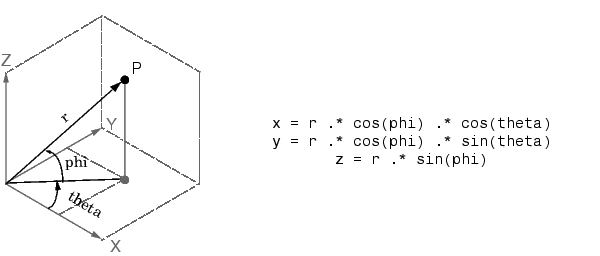MATLAB Function Referencesph2cart

Transform spherical coordinates to Cartesian

Syntax

• ```[x,y,z] = sph2cart(THETA,PHI,R)
```

Description

```[x,y,z] = sph2cart(THETA,PHI,R) ``` transforms the corresponding elements of spherical coordinate arrays to Cartesian, or xyz, coordinates. `THETA`, `PHI`, and `R` must all be the same size. `THETA` and `PHI` are angular displacements in radians from the positive x-axis and from the x-y plane, respectively.

Algorithm

The mapping from spherical coordinates to three-dimensional Cartesian coordinates is`cart2pol`, `cart2sph`, `pol2cart`